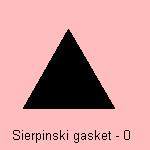## fractal

last updated: 2005-03-27

The Sierpinski gasket is a base motif fractal where the base is a triangle. After subdivision in 4 equal triangles the motif is to remove the middle triangle:The curve is also known as the Sierpinski triangle or as the Sierpinski triangle curve. It was Mandelbrot who first gave it the name of the Sierpinski gasket.

Waclaw Sierpinski (1882-1969), a Polish mathematician, described the construction to give an example of a curve simultaneously Cantorian and Jordanian, of which every point is a point of ramification.

The fractal dimension of the curve is equal to log 3/ log 2, i.e. about 1.58496 1).

A Sierpinski gasket constructed from resistors gives a simple network, that can be used to study  electrical conductivity, diffusive transport and thermodynamic properties 2).

notes

1) Fractal dimension = log N / log e, where N is the number of line segments and e the magnification.
For the Sierpinski gasket: N=3, e=2.

2) W.K. Ching, M. Erickson, P. Garik, P. Hickman, J. Jordan, S. Schwarzer, and L. Shorem Overcoming Resistance with Fractals: A New Way to Teach Elementary Circuits, in The Physics Teacher, Vol 32., No. 9 (December 1994), p546-551.